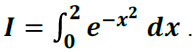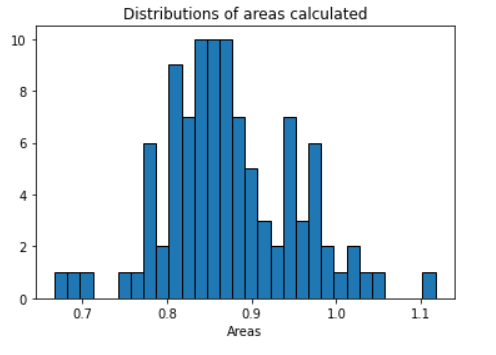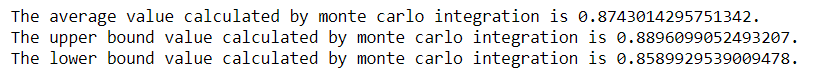# Monte-Carlo-Simulation

Monte Carlo Simulation to estimate the answer for an Integral in Python as well as Microsoft Excel

a. Built a simulation model in Python and Microsoft Excel to estimate the value of the integral,Using the limits of integration shown above and running the model for 100 iterations for estimating the answer for the exponential integral by iterating over each value of the variable to be estimated and the value being sampled off of a uniform distribution within the limits of a and b.

b. Plotted the resulting distribution of the calculated areas with the help of histogram function in matplotlib for 30 bins.c. Calculated the 95% confidence interval, along with the average, upper bound and lower bound for the answer of the integral.View Github